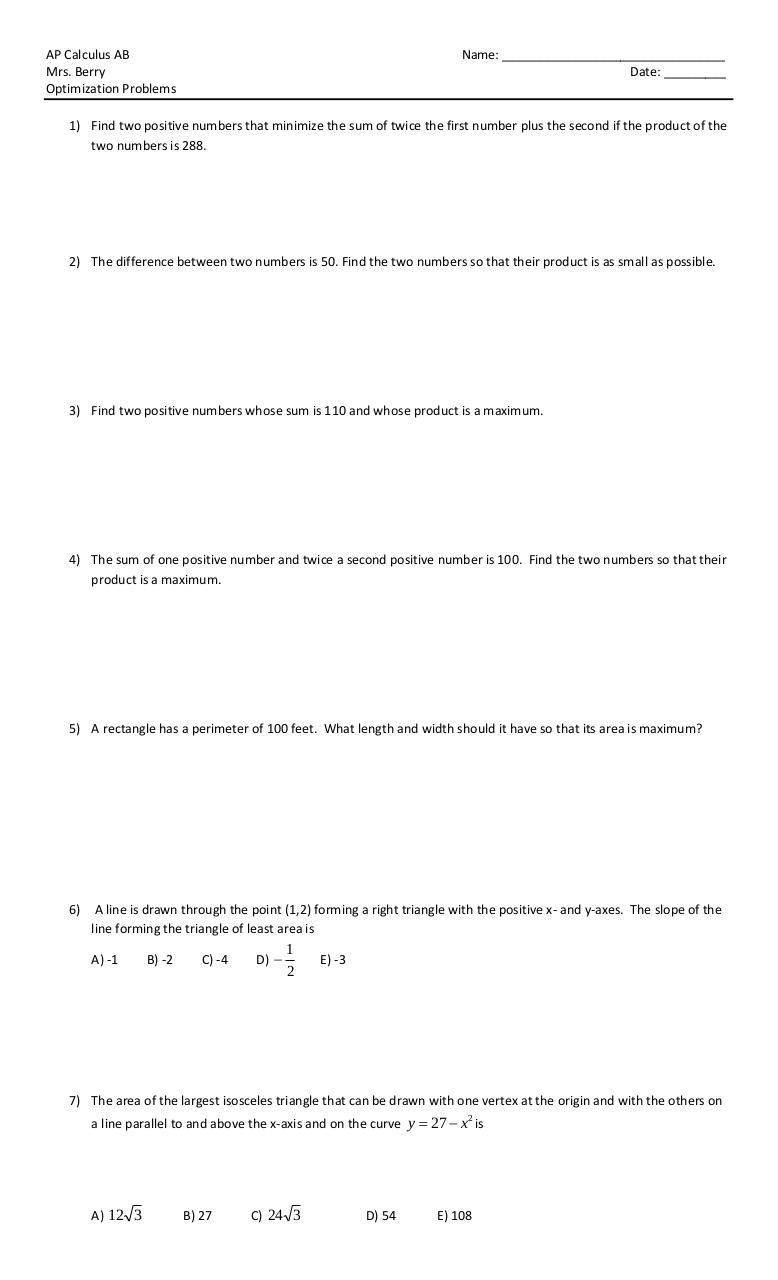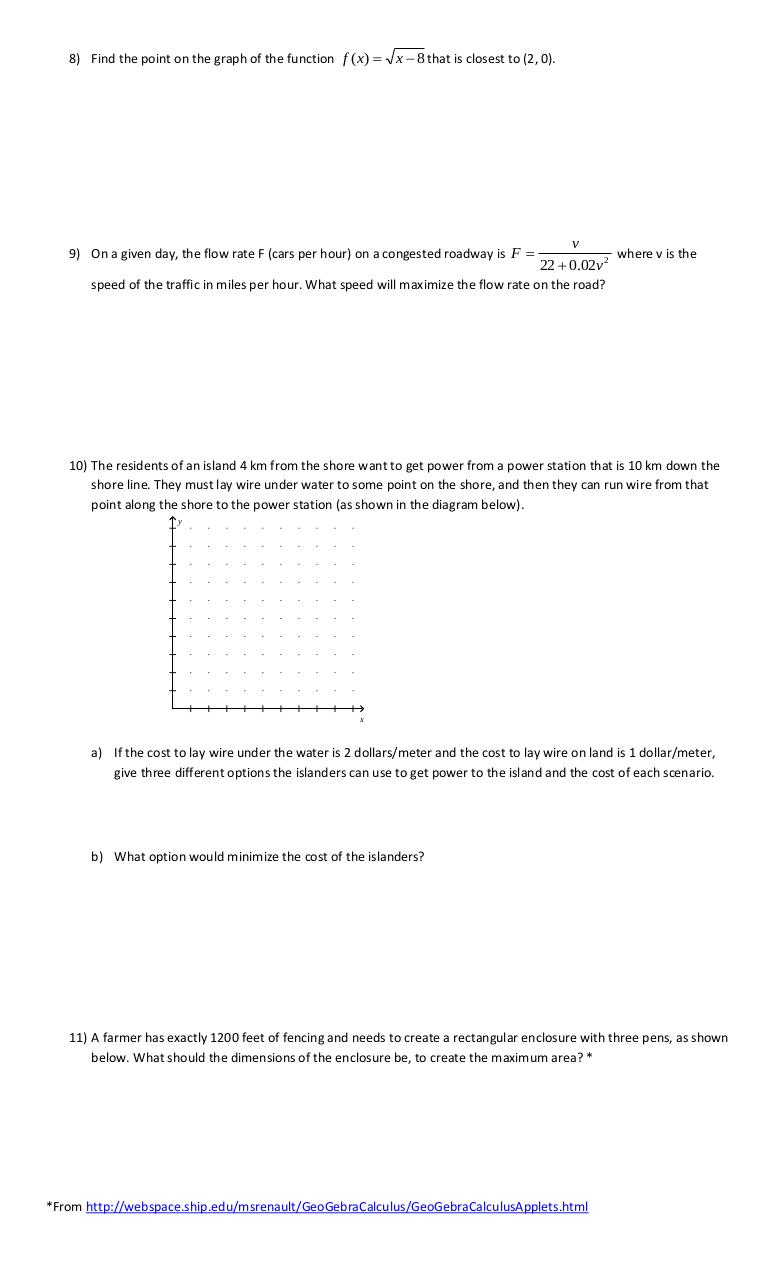# Optimization .pdf

### File information

Original filename: Optimization.pdf
Author: Berry Heather (13K430)

This PDF 1.5 document has been generated by MicrosoftÂ® Word 2010, and has been sent on pdf-archive.com on 16/01/2018 at 01:12, from IP address 47.17.x.x. The current document download page has been viewed 531 times.
File size: 120 KB (2 pages).
Privacy: public file

Optimization.pdf (PDF, 120 KB)

### Document preview

AP Calculus AB
Mrs. Berry
Optimization Problems

Name: ________________________________
Date: _________

1) Find two positive numbers that minimize the sum of twice the first number plus the second if the product of the
two numbers is 288.

2) The difference between two numbers is 50. Find the two numbers so that their product is as small as possible.

3) Find two positive numbers whose sum is 110 and whose product is a maximum.

4) The sum of one positive number and twice a second positive number is 100. Find the two numbers so that their
product is a maximum.

5) A rectangle has a perimeter of 100 feet. What length and width should it have so that its area is maximum?

6)

A line is drawn through the point (1,2) forming a right triangle with the positive x- and y-axes. The slope of the
line forming the triangle of least area is
A) -1

B) -2

C) -4

D) 

1
2

E) -3

7) The area of the largest isosceles triangle that can be drawn with one vertex at the origin and with the others on
a line parallel to and above the x-axis and on the curve y  27  x is
2

A) 12 3

B) 27

C) 24 3

D) 54

E) 108

8) Find the point on the graph of the function f ( x) 

x  8 that is closest to (2, 0).

9) On a given day, the flow rate F (cars per hour) on a congested roadway is F 

v
where v is the
22  0.02v 2

speed of the traffic in miles per hour. What speed will maximize the flow rate on the road?

10) The residents of an island 4 km from the shore want to get power from a power station that is 10 km down the
shore line. They must lay wire under water to some point on the shore, and then they can run wire from that
point along the shore to the power station (as shown in the diagram below).
y

x

a) If the cost to lay wire under the water is 2 dollars/meter and the cost to lay wire on land is 1 dollar/meter,
give three different options the islanders can use to get power to the island and the cost of each scenario.

b) What option would minimize the cost of the islanders?

11) A farmer has exactly 1200 feet of fencing and needs to create a rectangular enclosure with three pens, as shown
below. What should the dimensions of the enclosure be, to create the maximum area? *

*From http://webspace.ship.edu/msrenault/GeoGebraCalculus/GeoGebraCalculusApplets.html#### HTML Code

Copy the following HTML code to share your document on a Website or Blog

#### QR Code### Related keywords